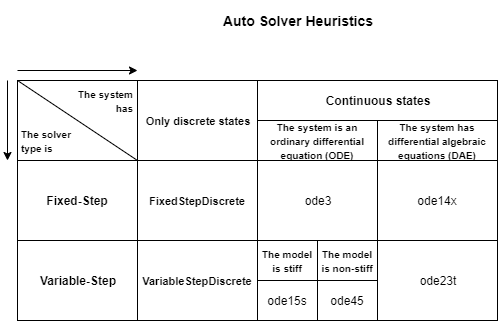## Select Solver Using Auto Solver

When you want Simulink® to select a solver for simulating the model, use auto solver. Auto solver chooses a suitable solver and sets the maximum step size of the simulation.

For new models, Simulink selects auto solver and sets the type to variable-step by default. For an existing model, you can use auto solver to select a solver.

1. Open `vdp` and click the solver link in the lower-right corner.2. In the Solver Information pane, click the View solver settings buttonto open the Solver pane of the model configuration parameters.

3. Under Solver selection, set Type to fixed or variable-step according to your preference and set Solver to `auto`.

4. When you simulate the model, auto solver selects a fixed-step or variable-step solver according to your preference and calculates the maximum step size it recommends. To see the results, open the Solver information pane.5. Click the Accept suggested settings buttonto apply the recommendations of auto solver. To select different settings, click the View solver settings button and make changes in the configuration parameters Solver pane.

This chart describes the selection process of the auto solver.• For Simscape™ Electrical™ models, auto solver selects `ode23tb`. These systems can have circuits with nonlinear models, especially circuit breakers and power electronics. Such nonlinear models require a stiff solver.

• If the number of continuous states in the model exceeds the `NumStatesForStiffnessChecking` value, auto solver uses `ode15s`. It does not calculate the stiffness of the model. The default value for this parameter is 1000. You can change this value using `set_param`.

• If the number of continuous states in the model is less than the `NumStatesForStiffnessChecking` value, auto solver calculates the stiffness of the model. A model is stiff if the stiffness exceeds the `StiffnessThreshold` value. The default value for this parameter is 1000. You can change this value using `set_param`.